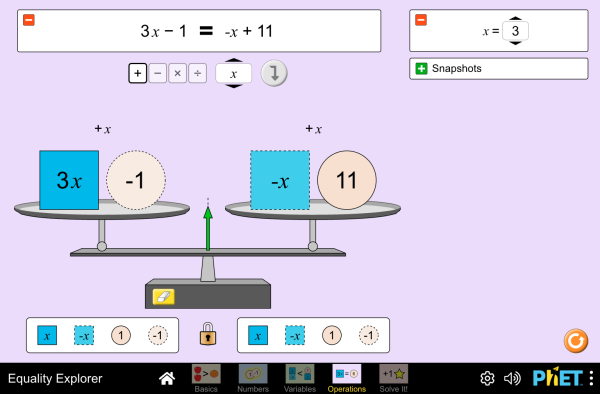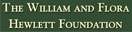PreuzimanjeUgrađivanje zatvorite Ugradite interaktivnu verziju ove simulacije Koristite ovaj HTML kod da ugradite interaktivnu verziju ove simulacije. Možete promijeniti širinu i visinu ugrađene simulacije tako što ćete doraditi "width"(širina) i "height" (visina) atribute u HTML kodu. Ugradite sliku koja će na klik pokrenuti simulaciju
Kliknite za pokretanje
Koristite ovaj HTML kod da biste prikazali sliku ekrana sa riječima "Kliknite za pokretanje". Rješavanje jednadžbi Nejednakosti Inverzne operacije PhET je podržan odi učitelja poput Vas.

### Teme

• Nejednakosti
• Inverzne operacije

### Opis

Explore what it means for a mathematical statement to be balanced or unbalanced by interacting with objects on a balance. Discover the rules for keeping it balanced. Collect stars by playing the game!

### Uzorak ciljeva učenja

• Solve and manipulate algebraic equations by applying properties of real numbers (in particular, the inverse properties of addition and multiplication) and properties of equality
• Solve and manipulate algebraic equations by substituting different values for a variable
• Use a balance model to solve an equation for an unknown, and justify your strategies for solving
• Solve an equation using only universal operations

### Usklađivanje standarda

#### Zajednička jezgra - Matematika

6.EE.B.5
Understand solving an equation or inequality as a process of answering a question: which values from a specified set, if any, make the equation or inequality true? Use substitution to determine whether a given number in a specified set makes an equation or inequality true.
6.EE.B.6
Use variables to represent numbers and write expressions when solving a real-world or mathematical problem; understand that a variable can represent an unknown number, or, depending on the purpose at hand, any number in a specified set.
7.EE.B.3
Solve multi-step real-life and mathematical problems posed with positive and negative rational numbers in any form (whole numbers, fractions, and decimals), using tools strategically. Apply properties of operations to calculate with numbers in any form; convert between forms as appropriate; and assess the reasonableness of answers using mental computation and estimation strategies. For example: If a woman making \$25 an hour gets a 10% raise, she will make an additional 1/10 of her salary an hour, or \$2.50, for a new salary of \$27.50. If you want to place a towel bar 9 3/4 inches long in the center of a door that is 27 1/2 inches wide, you will need to place the bar about 9 inches from each edge; this estimate can be used as a check on the exact computation.
7.EE.B.4
Use variables to represent quantities in a real-world or mathematical problem, and construct simple equations and inequalities to solve problems by reasoning about the quantities.
8.EE.C.7
Solve linear equations in one variable.
8.EE.C.7a
Give examples of linear equations in one variable with one solution, infinitely many solutions, or no solutions. Show which of these possibilities is the case by successively transforming the given equation into simpler forms, until an equivalent equation of the form x = a, a = a, or a = b results (where a and b are different numbers).
8.EE.C.8
Analyze and solve pairs of simultaneous linear equations.
HSA-CED.A.1
Create equations and inequalities in one variable and use them to solve problems. Include equations arising from linear and quadratic functions, and simple rational and exponential functions.
HSA-CED.A.3
Represent constraints by equations or inequalities, and by systems of equations and/or inequalities, and interpret solutions as viable or nonviable options in a modeling context. For example, represent inequalities describing nutritional and cost constraints on combinations of different foods.
HSA-REI.A.1
Explain each step in solving a simple equation as following from the equality of numbers asserted at the previous step, starting from the assumption that the original equation has a solution. Construct a viable argument to justify a solution method.
HSA-REI.B.3
Solve linear equations and inequalities in one variable, including equations with coefficients represented by letters.
Verzija 1.0.12

### Savjeti učiteljaPregled kontrole simulacije, pojednostavljenje modela i uvid u učenička razmišljanja ( PDF ).

### Aktivnosti predane od strane učitelja

Introducción a la igualdadDiana López HS
MS
Guided
HW
Lab
Mathematics
Balancing ActMichael Reynolds MS Guided Mathematics
What is EqualityDavid Wood HS
MS
Lab
Discuss
HW
Guided
Mathematics
Introduction to InequalitiesMarleene Buttice MS Guided Mathematics
Solving Multi-Step EquationsMeenakshi Raghavan MS Guided Mathematics
Middle School Math Sim AlignmentAmanda McGarry MS Other Mathematics
PRIMARIA: Alineación con programas de la SEP México (2011 y 2017)Diana López K-5
MS
HW
Demo
Lab
Guided
Discuss
Physics
Chemistry
Mathematics
Astronomy
Equality Explorer - 6th Grade intro to solving Trevor Gibson MS Guided Mathematics
Exploring Equations Akili Obika HS
MS
Guided Mathematics
Equação e balança Alison Sousa da Silva, Ana Carolina Costa Pereira MS Guided
Lab
Mathematics
Jezik Preuzmite ili pokrenite Savjeti
Arapski Sve العربيةمستكشف المساواة
Basque Sve EuskaraBerdintasun-esploratzailea
Bosanski Sve BosanskiIstraživač jednačina
Češki Sve češtinaPrůzkumník rovnosti
Danski Sve DanskLigevægts-visning
Engleski Sve EnglishEquality Explorer
Francuski Sve françaisExplorateur d'égalité
Grčki Sve ΕλληνικάΕξερευνητής εξισώσεων
Hindi Sve हिंदीसमानता का पता करें
Japanski Sve 日本語つり合いを作ろう
Kazakh Sve KazakhТеңсіздіктер шешімі
Kineski (pojednostavljen) Sve 中文 (中国)等式探索
Kineski (traditionalni) Sve 中文 (台灣)Equality Explorer_探索等式
Korejski Sve 한국어등식 연습하기
Letonski Sve LatviešuVienādību pētīšana
Mađarski Sve magyarEgyenlőségvizsgálat
Mongolian Sve Монгол (Монгол)Тэнцэтгэл Судлах
Nizozemski Sve NederlandsOntdek gelijkheid
Njemački Sve DeutschGleichheit schaffen
Poljski Sve polskiPoznajemy równania
Portugalski Sve portuguêsExplorador de Igualdades
Portugalski (Brazil) Sve português (Brasil)Explorador da Igualdade
Ruski Sve русскийРешение неравенств
Slovački Sve SlovenčinaSkúmanie rovnosti
Srpski Sve СрпскиИстраживач једначина
Španjolski Sve españolExplorador de IgualdadesŠpanjolski (Mexico) Sve español (México)Explorador de IgualdadesŠpanjolski (Peru) Sve español (Perú)Explorador de Igualdad
Švedski Sve svenskaUtforska likheter!
Talijanski Sve italianoEsplora le equazioni
Turski Sve TürkçeEşitlik Gezgini
Ukrajinski Sve українськаДослідник рівностейVijetnamski Sve Tiếng ViệtPhương trình

The new HTML5 sims can run on iPads and Chromebooks, as well as PC, Mac, and Linux systems.

iOS 12+ Safari

Android:
Not officially supported. If you are using the HTML5 sims on Android, we recommend using the latest version of Google Chrome.

Chromebook:
The HTML5 and Flash PhET sims are supported on all Chromebooks.
Chromebook compatible sims

Windows Systems:

Macintosh Systems:

Linux Systems:

Dizajnerski tim Biblioteke treće strane Zahvale
• Chris Malley (developer)
• Diana López Tavares
• Ariel Paul
• Kathy Perkins
• Argenta Price
• David Webb
• Mariah Hermsmeyer (graphic arts)
• Cheryl McCutchan (graphic arts)
• almond-0.2.9.js
• base64-js-1.2.0.js
• easing-equations-r12
• FileSaver-b8054a2.js
• font-awesome-4.5.0
• game-up-camera-1.0.0.js
• he-1.1.1.js
• himalaya-0.2.7.js
• jama-1.0.2
• jquery-2.1.0.js
• lodash-4.17.4.js
• pegjs-0.7.0.js
• seedrandom-2.4.2.js
• text-2.0.12.js
• TextEncoderLite-3c9f6f0.js
• Tween-r12.js